Customizing Chunk Options

When generating a HTML file from R Markdown, it is first processed with the knitr package. With this tool-chain, the wide array of knitr options can be used to customize how chunks appear in your document. These are explained at length on the knitr website and some examples of commonly used options are provided below. Note that the default value for each option is shown in the description.

Source Code Display

echo: (TRUE; logical or numeric) whether to include R source code in the output file; besides TRUE/FALSE which completely turns on/off the source code, we can also use a numeric vector to select which R expression(s) to echo in a chunk, e.g. echo=2:3 means only echo the 2nd and 3rd expressions, and echo=-4 means to exclude the 4th expression.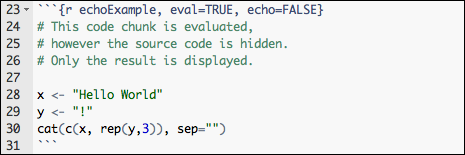## Hello World!!!

results: ('markup'; character) takes three possible values:

• markup: mark up the results using the output hook, e.g. put results in a special LaTeX environment.
• asis: output as-is, i.e., write raw results from R into the output document.
• hide hide results; this option only applies to normal R output (not warnings, messages or errors).
• note markup and asis are equivalent to verbatim and tex in Sweave respectively (you can still use the latter two, but they can be misleading, e.g., verbatim does not really mean verbatim in R, and tex seems to be restricted to LaTeX).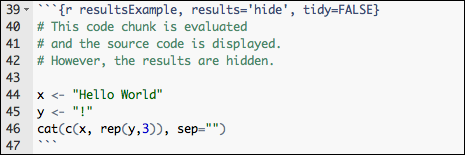# This code chunk is evaluated
# and the source code is displayed.
# However, the results are hidden.

x <- "Hello World"
y <- "!"
cat(c(x, rep(y,3)), sep="")

Plots

fig.width, fig.height: (both are 7; numeric) width and height of the plot, to be used in the graphics device (in inches) and have to be numeric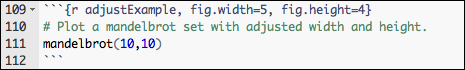# Plot a mandelbrot set with adjusted width and height.
mandelbrot(10, 10)Code Evaluation

eval: (TRUE; logical) whether to evaluate the code chunk.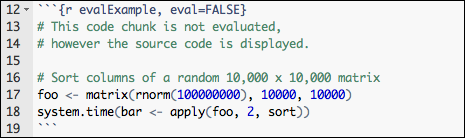# This code chunk is not evaluated, however the source code is displayed.

# Sort columns of a random 10,000 x 10,000 matrix
foo <- matrix(rnorm(1e+08), 10000, 10000)
system.time(bar <- apply(foo, 2, sort))

Code Decoration

prompt: (FALSE; logical) whether to add the prompt characters in the R code (see prompt and continue in ?base::options; note that adding prompts can make it difficult for readers to copy R code from the output, so prompt=FALSE may be a better choice.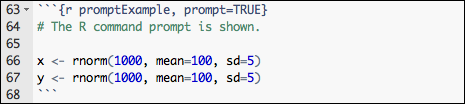> # The R command prompt is shown.
>
> x <- rnorm(1000, mean = 100, sd = 5)
> y <- rnorm(1000, mean = 100, sd = 5)